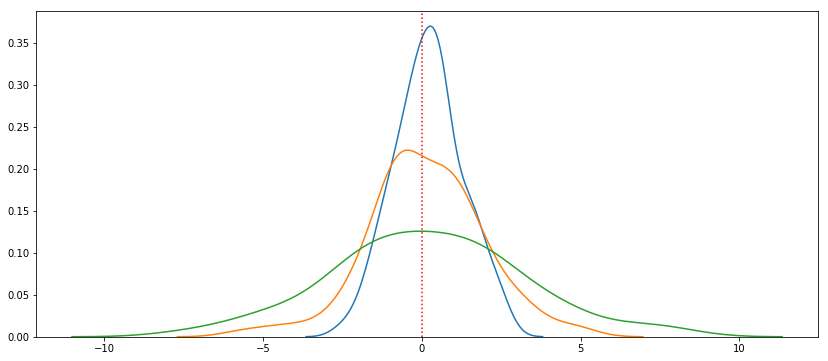# Intro to Statistics

Last updated: January 13th, 2020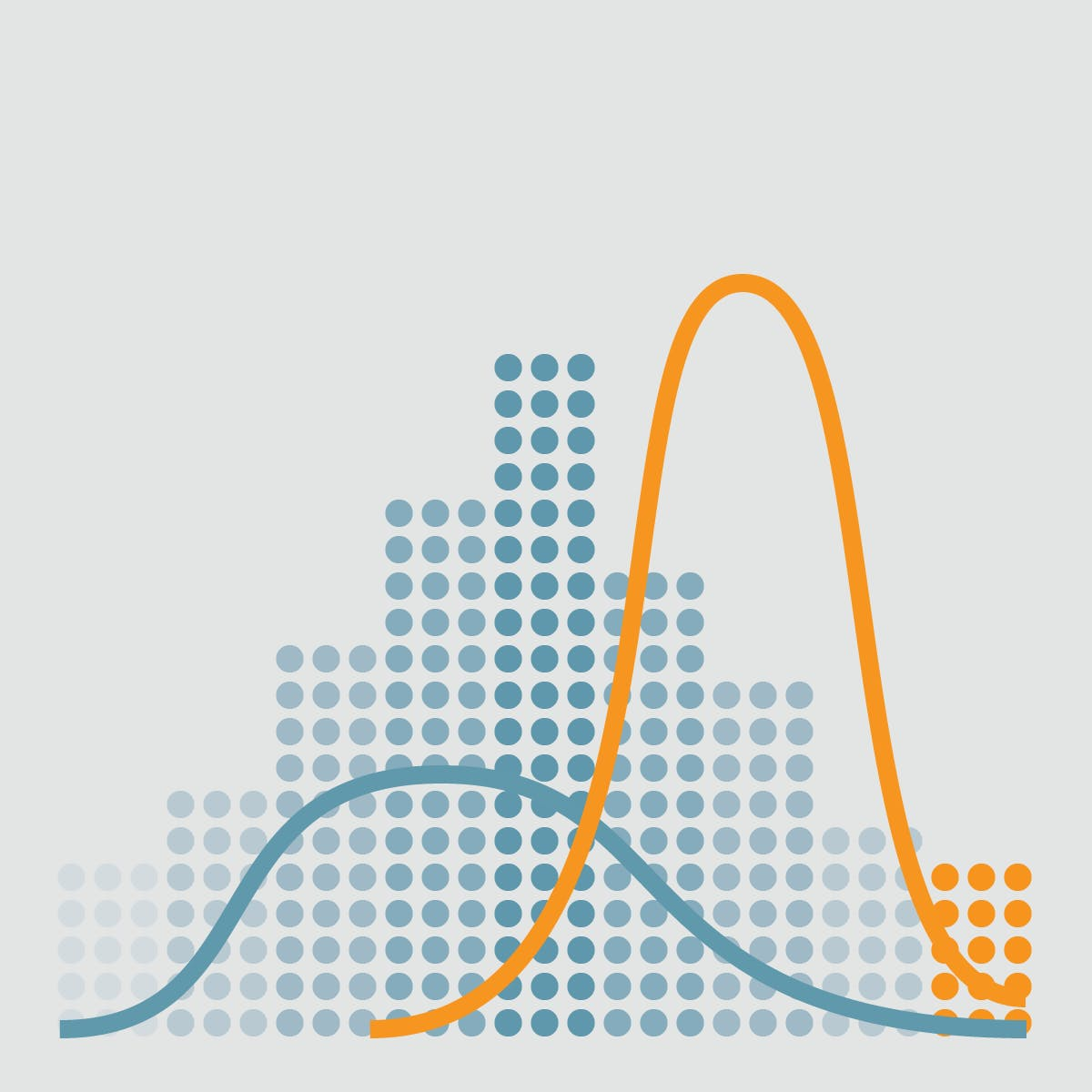# Intro to Statistics¶

Quick overview of basic estimators.## Hands on!¶

In :
import numpy as np
import pandas as pd
import matplotlib.pyplot as plt
import seaborn as sns

%matplotlib inline## Probability distribution fitting¶

The aim of distribution fitting is to predict the probability or to forecast the frequency of occurrence of the magnitude of the phenomenon in a certain interval.

We can understand data showing its distribution.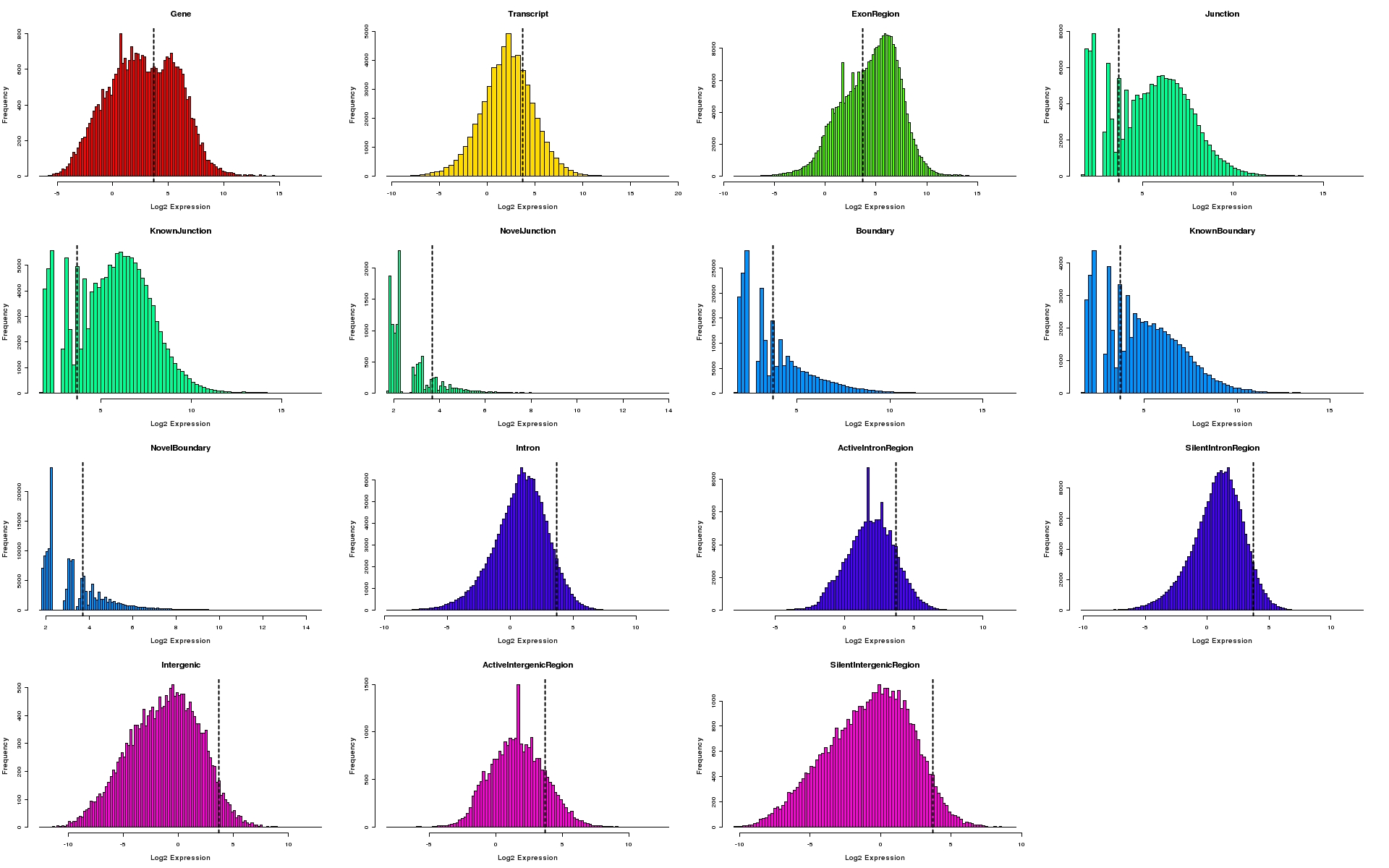## Basic estimators¶

We'll try to explain basic estimators without going deeper into difficult formulas yet.

Mean, median, standard deviation, range, ...

Let's look at an example:Suppose we have many baby duck families:

• Duck family 1: 5 baby ducks
• Duck family 2: 1 baby ducks
• Duck family 3: 3 baby ducks
• Duck family 4: 5 baby ducks
• Duck family 5: 2 baby ducks
• Duck family 6: 4 baby ducks
• Duck family 7: 22 baby ducks
In :
ducks = [5, 1, 3, 5, 2, 4, 22]

ducks

Out:
[5, 1, 3, 5, 2, 4, 22]

### Mean¶

The mean is used to summarize a data set. It is a measure of the center of a data set.

We can think of the mean as the number of baby ducks each mamma duck would have if they were equally distributed among all the mamma ducks.

In :
ducks

Out:
[5, 1, 3, 5, 2, 4, 22]
In :
ducks_sum = 5 + 1 + 3 + 5 + 2 + 4 + 22

ducks_sum

Out:
42
In :
mean = ducks_sum / len(ducks)

mean

Out:
6.0
In :
np.mean(ducks)

Out:
6.0
In :
plt.figure(figsize=(12,6))

plt.bar(["Family %s" % n for n in range(1, len(ducks)+1)], ducks)

# Mean line
plt.axhline(np.mean(ducks), color='r', linestyle=':', linewidth=2)

Out:
<matplotlib.lines.Line2D at 0x7f395ff35518>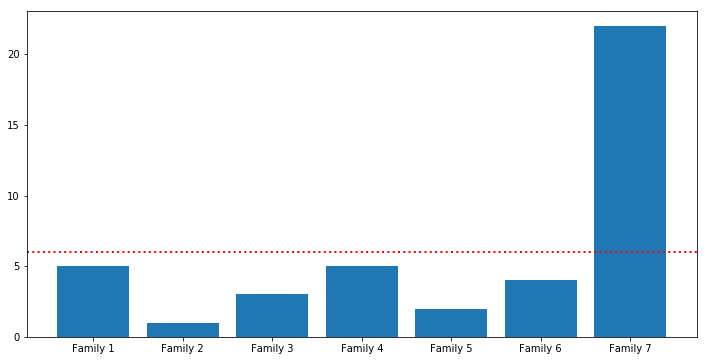### Median¶

The median is the the middle number; found by ordering all data points and picking out the one in the middle (or if there are two middle numbers, taking the mean of those two numbers).

In :
ducks

Out:
[5, 1, 3, 5, 2, 4, 22]

First we'll order that values:

$$1, 2, 3, 4, 5, 5, 22$$

Then we get the middle of all the values: 4

And that is our median.

In :
np.median(ducks)

Out:
4.0
In :
plt.figure(figsize=(12,6))

plt.bar(["Family %s" % n for n in range(1, len(ducks)+1)], ducks)

# Mean line
plt.axhline(np.mean(ducks), color='r', linestyle=':', linewidth=2)

# Median line
plt.axhline(np.median(ducks), color='orange', linestyle=':', linewidth=2)

Out:
<matplotlib.lines.Line2D at 0x7f395feeb1d0>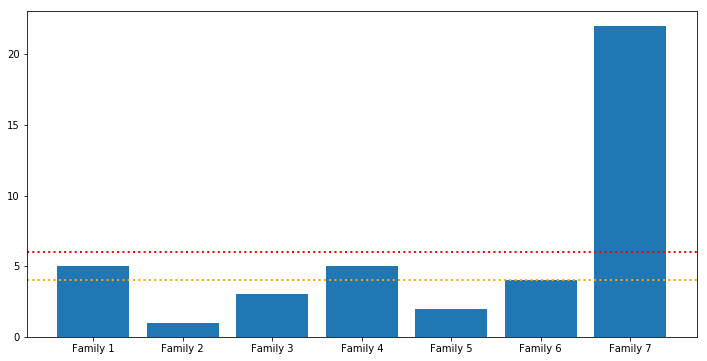In :
plt.figure(figsize=(12,6))

pd.Series(ducks).value_counts().sort_index().plot(kind='bar')

Out:
<matplotlib.axes._subplots.AxesSubplot at 0x7f395e51cf98>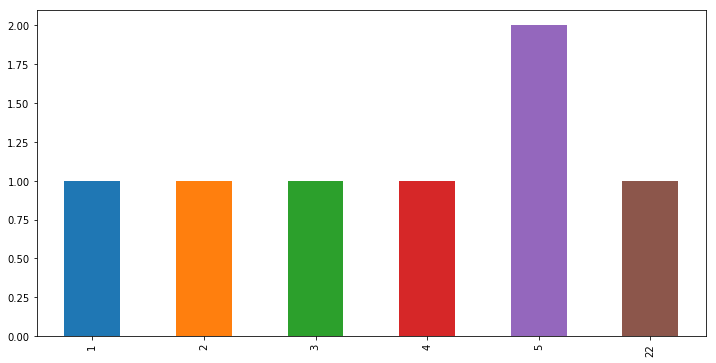In :
plt.figure(figsize=(12,6))

pd.Series(ducks).value_counts().sort_index().reindex(list(range(23))).fillna(0).plot(kind='bar')

Out:
<matplotlib.axes._subplots.AxesSubplot at 0x7f395f0af5f8>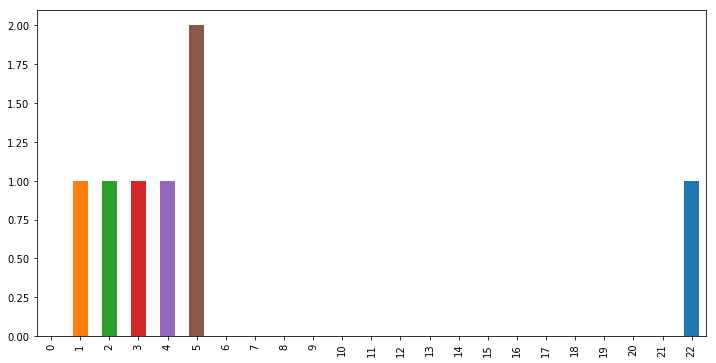In :
plt.figure(figsize=(14,6))

sns.distplot(ducks, hist=False)

# Mean line
plt.axvline(np.mean(ducks), color='r', linestyle=':', linewidth=2)

# Median line
plt.axvline(np.median(ducks), color='orange', linestyle=':', linewidth=2)

Out:
<matplotlib.lines.Line2D at 0x7f5e3427b160>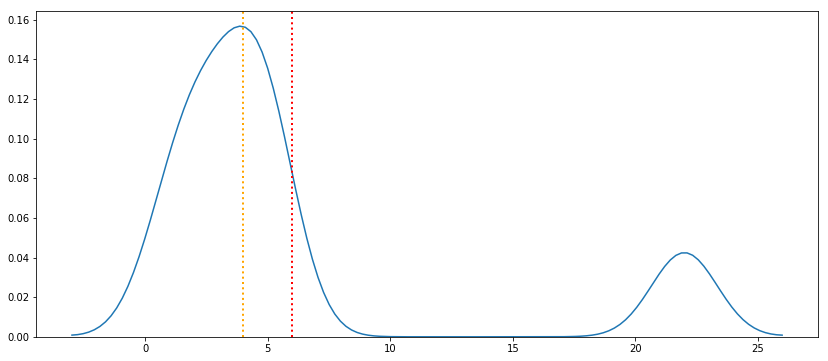### Mode¶

The mode is the most frequent number—that is, the number that occurs the highest number of times.

In :
ducks

Out:
[5, 1, 3, 5, 2, 4, 22]
In :
plt.figure(figsize=(12,6))

pd.Series(ducks).value_counts().sort_index().reindex(list(range(23))).fillna(0).plot(kind='bar')

Out:
<matplotlib.axes._subplots.AxesSubplot at 0x7f395f151828>In :
from statistics import mode

mode(ducks)

Out:
5

### Comparing ducks with dogs¶

Now we'll compare our duck families to dog families:

• Dog family 1: 6 puppies
• Dog family 2: 3 puppies
• Dog family 3: 7 puppies
• Dog family 4: 8 puppies
• Dog family 5: 4 puppies
• Dog family 6: 6 puppies
• Dog family 7: 8 puppies
In :
dogs = [6, 3, 7, 8, 4, 6, 8]

dogs

Out:
[6, 3, 7, 8, 4, 6, 8]

Let's analyze puppies mean per family:

In :
dogs_sum = 6 + 3 + 7 + 8 + 4 + 6 + 8

dogs_sum

Out:
42
In :
dogs_sum / len(dogs)

Out:
6.0
In :
np.mean(dogs)

Out:
6.0
In :
np.mean(ducks)

Out:
6.0
In :
plt.figure(figsize=(12,6))

pd.Series(dogs).value_counts().sort_index().reindex(list(range(max(dogs) + 1))).fillna(0).plot(kind='bar')

Out:
<matplotlib.axes._subplots.AxesSubplot at 0x7f395f29c630>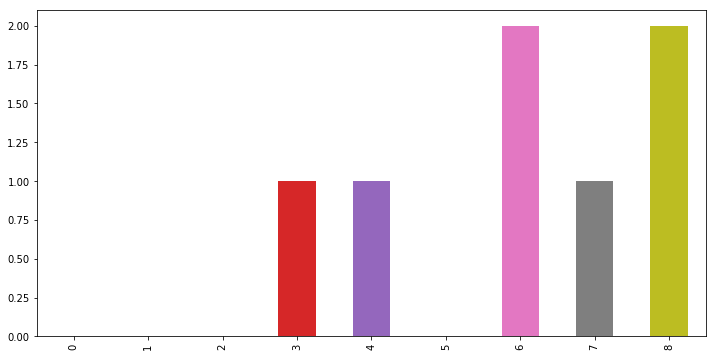We have the same mean on ducks and ducks, but both families has the same dispersion of babies?

### Range¶

The range is the difference between the lowest and highest values:

In :
print(min(ducks), '---', max(ducks))

1 --- 22

In :
ducks_range = max(ducks) - min(ducks)

ducks_range

Out:
21
In :
print(min(dogs), '---', max(dogs))

3 --- 8

In :
dogs_range = max(dogs) - min(dogs)

dogs_range

Out:
5

We see here that ducks has four times higher dispersion than dogs!## Why stats?¶

The objective of learning stats is to quickly understand the data you're working with, by just looking at the estimators described above. This requires experience, but after some time you'll be able to "picture" the shape of your data in your brain by just looking at these numbers.

All these estimators will affect "the shape" of the distribution, and that will have different impacts in your data:

• Mean
• Median
• Mode
• Standard deviation
• Percentiles
• Ranges
• IQR

We saw in the previous example, how two "distributions", ducks and dogs, had the same mean (6) but the numbers looked completely different. Duck families ranged from 1 to 22 while dogs ranged from 3 to 8. This will have a deep impact on your data analysis tasks later. For example, while cleaning data, if you find a dog family with 18 puppies, you'll probably need to investigate a little bit more (18 babies sounds unrealistic for dogs).

Let's see now how these different estimators will change the shape of the distributions.

### Altering the Mean¶

In :
plt.figure(figsize=(14,6))

sns.distplot(np.random.normal(0, 1, 100), hist=False)
plt.axvline(0, linestyle=':') # mean

sns.distplot(np.random.normal(2, 1, 100), hist=False)
plt.axvline(2, color='orange', linestyle=':') # mean

sns.distplot(np.random.normal(-2, 1, 100), hist=False)
plt.axvline(-2, color='green', linestyle=':') # mean

Out:
<matplotlib.lines.Line2D at 0x7f5e33af6438>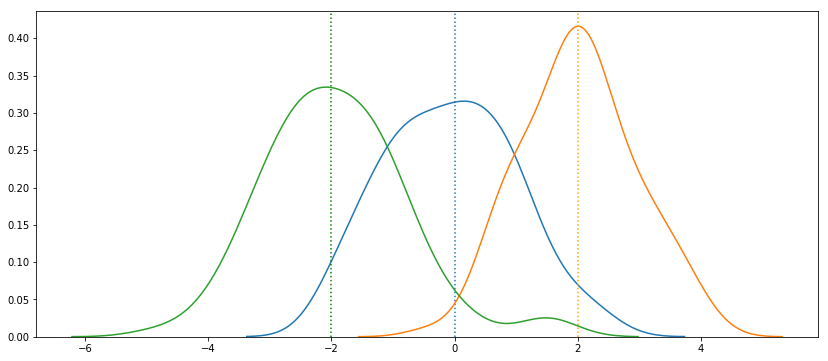### Altering the Standard Deviation¶

In :
plt.figure(figsize=(14,6))

data_1 = np.random.normal(0, 1, 100)
data_2 = np.random.normal(0, 2, 100)
data_3 = np.random.normal(0, 3, 100)

sns.distplot(data_1, hist=False)
sns.distplot(data_2, hist=False)
sns.distplot(data_3, hist=False)

plt.axvline(0, color='r', linestyle=':') # mean

Out:
<matplotlib.lines.Line2D at 0x7f5e33b1cf60>